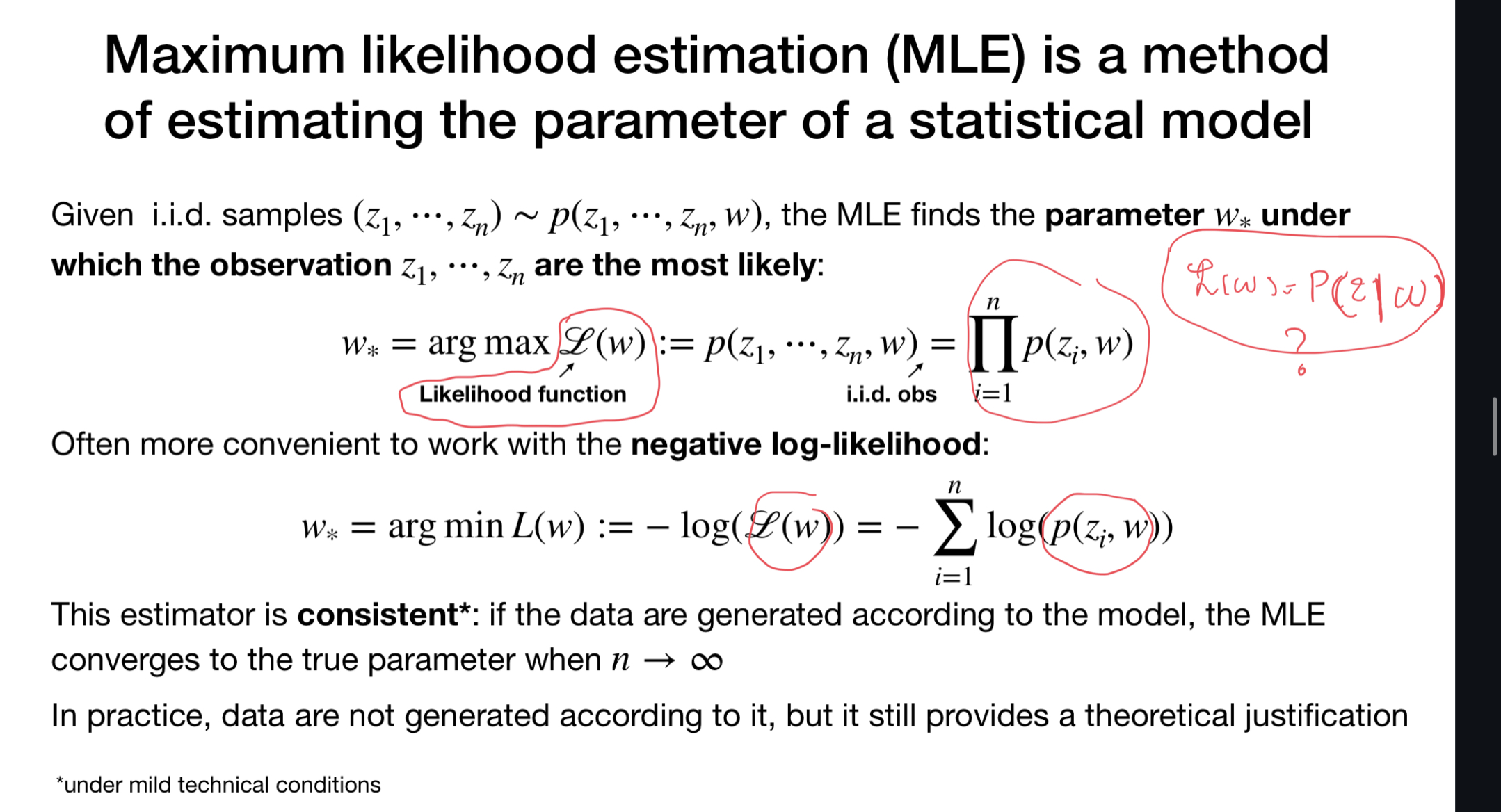### The likelihood function

I am confused by this slide. Isn’t the likelihood function is the probability of the observation given the parameter? Why do we have joint distribution as the likelihood in this slide?Top comment

It is just a notation. Only in bayesian statistics do parameters have distributions. Here we don't mean the joint distribution, only that the distribution is parametrized by $$w$$ (a semicolon ; should've been used or $$\mid$$ ), but I reiterate it is just a notation.

Page 1 of 1